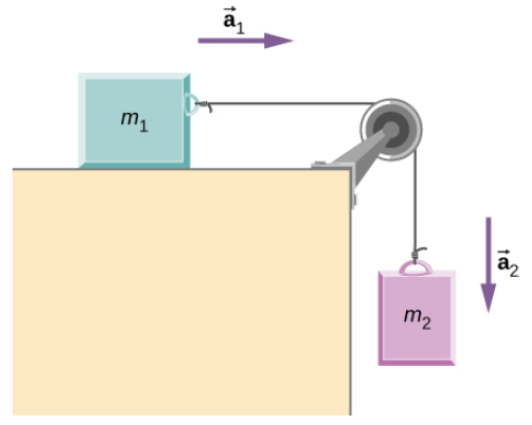# Problem: Two blocks are connected by a massless rope as shown below. The mass of the block on the table is 4.0 kg and the hanging mass is 1.0 kg. The table and the pulley are frictionless. (a) Find the acceleration of the system. (b) Find the tension in the rope. (c) Find the speed with which the hanging mass hits the floor if it starts from rest and is initially located 1.0 m from the floor.

###### FREE Expert Solution

Newton's second law:

$\overline{){\mathbf{\Sigma }}{\mathbf{F}}{\mathbf{=}}{\mathbf{m}}{\mathbf{a}}}$

Uniform accelerated motion (UAM) equations, a.k.a. "kinematics equations":

(a)

Applying Newton's second law to the block on the table:

ΣF = ma

T = m1a1

Applying Newton's second law to the hanging block: Tension pulls up,  weight is directed downward, and the acceleration is directed downward.

93% (386 ratings)###### Problem Details

Two blocks are connected by a massless rope as shown below. The mass of the block on the table is 4.0 kg and the hanging mass is 1.0 kg. The table and the pulley are frictionless. (a) Find the acceleration of the system. (b) Find the tension in the rope. (c) Find the speed with which the hanging mass hits the floor if it starts from rest and is initially located 1.0 m from the floor.